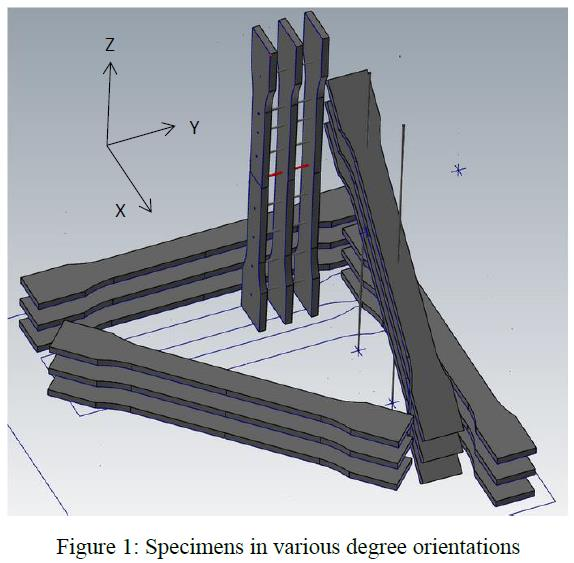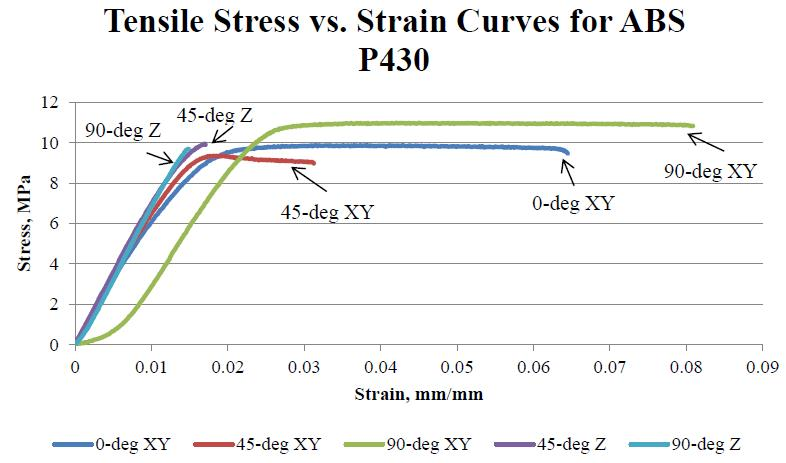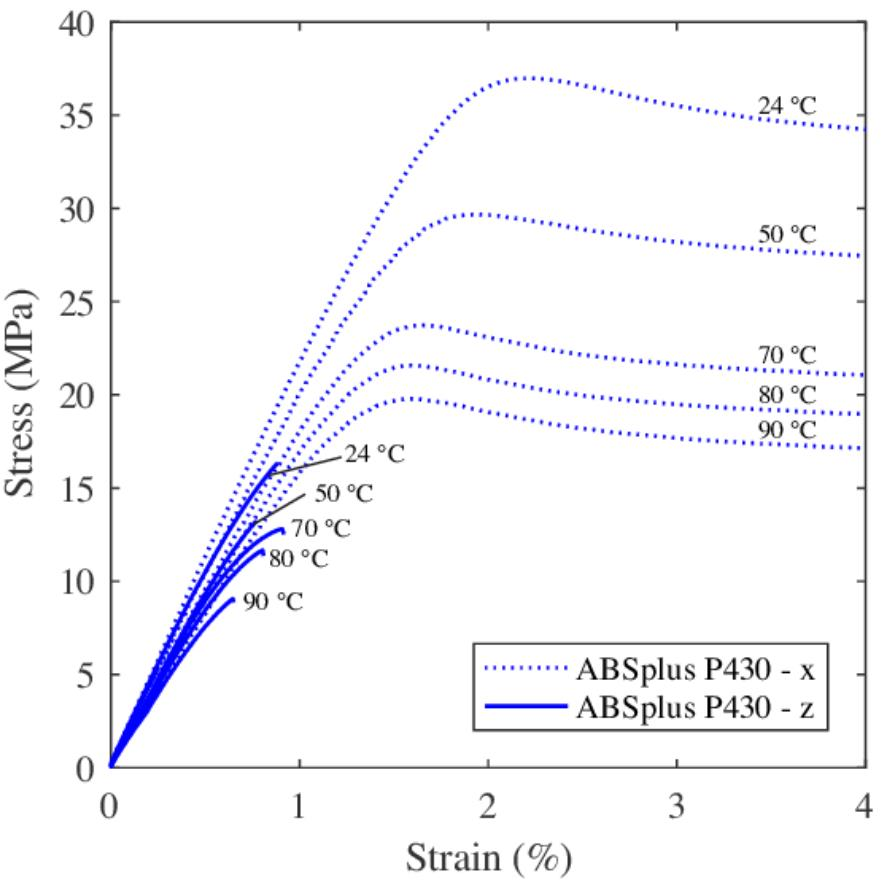peteroznewman
Subscriber

Hello Kahroba,

I'm guessing that you are 3D printing this structure. Here is a paper studying the tensile strength of ABS P430 material when printed in a 3D printer. There is a large difference in elongation at break depending on the direction of stress relative to the direction the material was printed in.

Below is the directions in which the test samples were printed, and the tensile stress was applied along the length of those test samples.Here is Figure 4 from that paper.What you can see from the plot above is that the point of fracture for material with tension along the Z axis of printing is at a strain of  about 1.5% but the point of fracture for material with tension along the 90-deg XY direction is over 8% strain.

I assume you are printing your structure layer by layer with the Z direction up, and will therefore avoid stress in the weakest Z direction. But that still leaves the material elongation varying between about 3 to 8 % total strain.

It does look like a simple Bilinear Kinematic Hardening Plasticity model with a zero Tangent Modulus will take that material past yield.  But all the above data is not for the ABS Plus.

I found a thesis that had ABS Plus P430 and that showed a yield strength significantly higher than the 10 MPa shown above. Here is the tensile stress strain curve from that thesis.Again, ignoring stress in the z direction, the yield in the x direction is over 30 MPa at room temperature, which is consistent with the data you found. This graph shows strain up to 4%, but it doesn't seem to show the point of fracture, which is a critical value to determine when a simulation model goes from simulating reality to going past the point of fracture and starting to create false information. Let's assume that 6% from your reference is the Total Strain at which fracture will occur.

When you say you want to simulate 50% strain, you mean that measured on the height of the cube of the structure, so if the structure is 50 mm tall, you want to squash it to 25 mm tall.

Build a model with a displacement of -25 mm in the Y axis, with the plasticity material defined, and plot maximum Total Strain vs. displacement and also plot Reaction Force for your energy calculation. The ANSYS model will only be valid up to the point when the maximum Total Strain reaches 6%. After that point, some area of the model would have fractured in a physical test of a real sample, but the ANSYS model does not fracture. The material just keeps on stretching. Therefore, the Reaction Force for the displacement past the point when the Total Strain reached 6% will not represent reality and will be larger than a physical sample from that displacement onward.

Good luck on the next stage of your model building.

Regards,

Peter